# ML Aggarwal Class 10 Solutions for ICSE Maths Chapter 11 Section Formula Ex 11

## ML Aggarwal Class 10 Solutions for ICSE Maths Chapter 11 Section Formula Ex 11

ML Aggarwal Class 10 Solutions for ICSE Maths Chapter 11 Section Formula Ex 11

Question 1.
Find the co-ordinates of the mid-point of the line segments joining the following pairs of points:
(i) (2, -3), ( -6, 7)
(ii) (5, -11), (4, 3)
(iii) (a + 3, 5b), (2a – 1, 3b + 4)
Solution:Question 2.
The co-ordinates of two points A and B are (-3, 3) and (12, -7) respectively. P is a point on the line segment AB such that AP : PB = 2 : 3. Find the co-ordinates of P.
Solution:Question 3.
P divides the distance between A (-2, 1) and B (1, 4) in the ratio of 2 : 1. Calculate the co-ordinates of the point P.
Solution:Question 4.
(i) Find the co-ordinates of the points of trisection of the line segment joining the point (3, -3) and (6, 9).
(ii) The line segment joining the points (3, -4) and (1, 2) is trisected at the points P and Q. If the coordinates of P and Q are (p, – 2) and $$\left( \frac { 5 }{ 3 } ,q \right)$$ respectively, find the values of p and q.
Solution: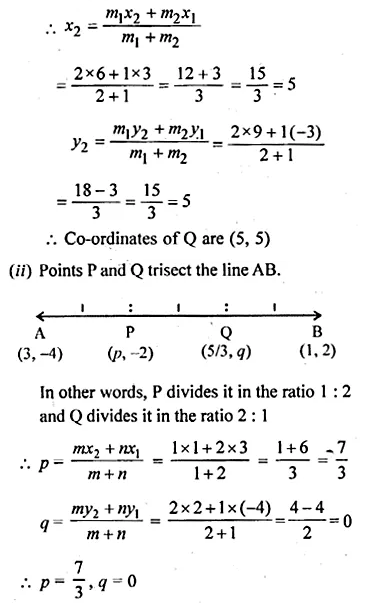Question 5.
(i) The line segment joining the points A (3, 2) and B (5, 1) is divided at the point P in the ratio 1 : 2 and it lies on the line 3x – 18y + k = 0. Find the value of k.
(ii) A point P divides the line segment joining the points A (3, -5) and B (-4, 8) such that $$\frac { AP }{ PB } =\frac { k }{ 1 }$$ If P lies on the line x + y = 0, then find the value of k.
Solution:Question 6.
Find the coordinates of the point which is three-fourths of the way from A (3, 1) to B (-2, 5).
Solution:Question 7.
Point P (3, -5) is reflected in P’ in the x-axis. Also, P on reflection in the y-axis is mapped as P”.
(i) Find the co-ordinates of P’ and P”.
(ii) Compute the distance P’ P”.
(iii) Find the middle point of the line segment P’ P”.
(iv) On which co-ordinate axis does the middle point of the line segment P P” lie?
Solution:Question 8.
Use graph paper for this question. Take 1 cm = 1 unit on both axes. Plot the points A(3, 0) and B(0, 4).
(i) Write down the co-ordinates of A1, the reflection of A in the y-axis.
(ii) Write down the co-ordinates of B1, the reflection of B in the x-axis.
(iii) Assign the special name to the quadrilateral ABA1B1.
(iv) If C is the midpoint is AB. Write down the co-ordinates of the point C1, the reflection of C in the origin.
(v) Assign the special name to quadrilateral ABC1B1.
Solution:Question 9.
The line segment joining A (-3, 1) and B (5, -4) is a diameter of a circle whose centre is C. find the co-ordinates of the point C. (1990)
Solution:Question 10.
The mid-point of the line segment joining the points (3m, 6) and (-4, 3n) is (1, 2m – 1). Find the values of m and n.
Solution:Question 11.
The co-ordinates of the mid-point of the line segment PQ are (1, -2). The co-ordinates of P are (-3, 2). Find the co-ordinates of Q.(1992)
Solution:Question 12.
AB is a diameter of a circle with centre C (-2, 5). If point A is (3, -7). Find:
(i) the length of radius AC.
(ii) the coordinates of B.
Solution:Question 13.
Find the reflection (image) of the point (5, -3) in the point (-1, 3).
Solution:Question 14.
The line segment joining A $$\left( -1,\frac { 5 }{ 3 } \right)$$ the points B (a, 5) is divided in the ratio 1 : 3 at P, the point where the line segment AB intersects y-axis. Calculate
(i) the value of a
(ii) the co-ordinates of P. (1994)
Solution:Question 15.
The point P (-4, 1) divides the line segment joining the points A (2, -2) and B in the ratio of 3 : 5. Find the point B.
Solution:Question 16.
(i) In what ratio does the point (5, 4) divide the line segment joining the points (2, 1) and (7 ,6) ?
(ii) In what ratio does the point (-4, b) divide the line segment joining the points P (2, -2), Q (-14, 6) ? Hence find the value of b.
Solution:Question 17.
The line segment joining A (2, 3) and B (6, -5) is intercepted by the x-axis at the point K. Write the ordinate of the point k. Hence, find the ratio in which K divides AB. Also, find the coordinates of the point K.
Solution:Question 18.
If A (-4, 3) and B (8, -6)
(i) find the length of AB.
(ii) in what ratio is the line joining AB, divided by the x-axis? (2008)
Solution: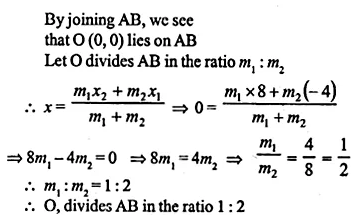Question 19.
(i) Calculate the ratio in which the line segment joining (3, 4) and(-2, 1) is divided by the y-axis.
(ii) In what ratio does the line x – y – 2 = 0 divide the line segment joining the points (3, -1) and (8, 9)?
Also, find the coordinates of the point of division.
Solution: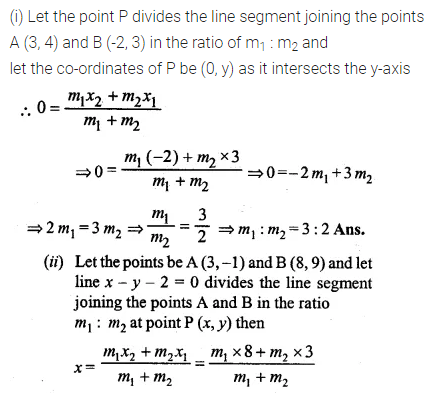Question 20.
Given a line segment AB joining the points A (-4, 6) and B (8, -3). Find:
(i) the ratio in which AB is divided by the y-axis.
(ii) find the coordinates of the point of intersection.
(iii)the length of AB.
Solution: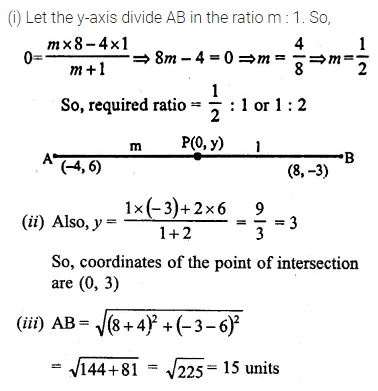Question 21.
(i) Write down the co-ordinates of the point P that divides the line joining A (-4, 1) and B (17, 10) in ratio 1 : 2.
(ii)Calculate the distance OP where O is the origin.
(iii)In what ratio does the y-axis divide the line AB?
Solution: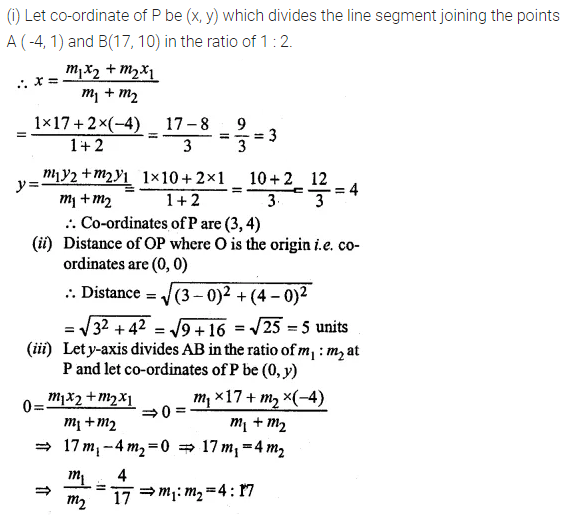Question 22.
Calculate the length of the median through the vertex A of the triangle ABC with vertices A (7, -3), B (5, 3) and C (3, -1)
Solution:Question 23.
Three consecutive vertices of a parallelogram ABCD are A (1, 2), B (1, 0) and C (4, 0). Find the fourth vertex D.
Solution:Question 24.
If the points A (-2, -1), B (1, 0), C (p, 3) and D (1, q) from a parallelogram ABCD, find the values of p and q.
Solution:Question 25.
If two vertices of a parallelogram are (3, 2) (-1, 0) and its diagonals meet at (2, -5), find the other two vertices of the parallelogram.
Solution: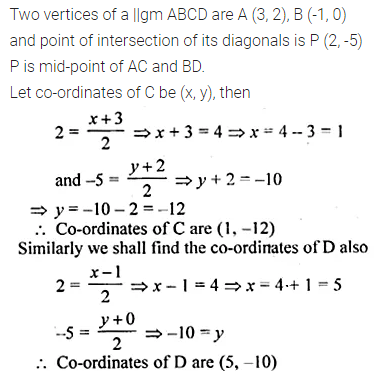Question 26.
Prove that the points A (-5, 4), B (-1, -2) and C (5, 2) are the vertices of an isosceles right-angled triangle. Find the coordinates of D so that ABCD is a square.
Solution:Question 27.
Find the third vertex of a triangle if its two vertices are (-1, 4) and (5, 2) and the midpoint of one side is (0, 3).
Solution:Question 28.
Find the coordinates of the vertices of the triangle the middle points of whose sides are $$\left( 0,\frac { 1 }{ 2 } \right) ,\left( \frac { 1 }{ 2 } ,\frac { 1 }{ 2 } \right) and\left( \frac { 1 }{ 2 } ,0 \right)$$
Solution:Question 29.
Show by section formula that the points (3, -2), (5, 2) and (8, 8) are collinear.
Solution:Question 30.
Find the value of p for which the points (-5, 1), (1, p) and (4, -2) are collinear.
Solution:Question 31.
A (10, 5), B (6, -3) and C (2, 1) are the vertices of triangle ABC. L is the midpoint of AB, M is the mid-point of AC. Write down the co-ordinates of L and M. Show that LM = $$\\ \frac { 1 }{ 2 }$$ BC.
Solution: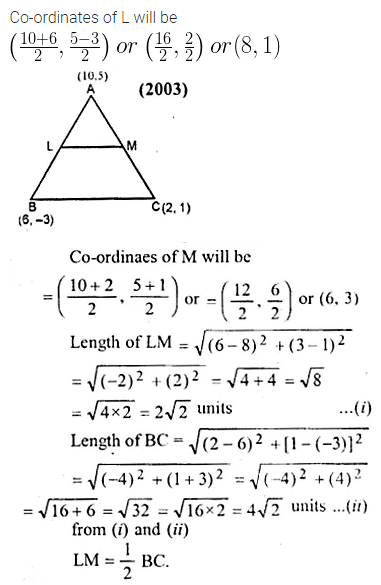Question 32.
A (2, 5), B (-1, 2) and C (5, 8) are the vertices of a triangle ABC. P and.Q are points on AB and AC respectively such that AP : PB = AQ : QC = 1 : 2.
(i) Find the co-ordinates of P and Q.
(ii) Show that PQ = $$\\ \frac { 1 }{ 3 }$$ BC.
Solution:Question 33.
The mid-point of the line segment AB shown in the adjoining diagram is (4, -3). Write down die co-ordinates of A and B.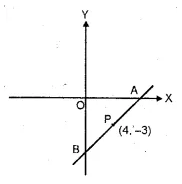Solution:Question 34.
Find the co-ordinates of the centroid of a triangle whose vertices are A (-1, 3), B(1, -1) and C (5, 1) (2006)
Solution: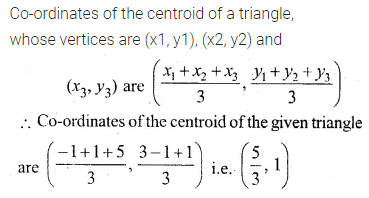Question 35.
Two vertices of a triangle are (3, -5) and (-7, 4). Find the third vertex given that the centroid is (2, -1).
Solution: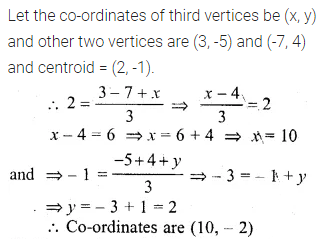Question 36.
The vertices of a triangle are A (-5, 3), B (p, -1) and C (6, q). Find the values of p and q if the centroid of the triangle ABC is the point (1, -1).
Solution: